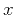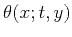Asymptotic pseudounitary stacking operatorsNext: Conclusions Up: EXAMPLES Previous: Velocity Transform

## Offset Continuation and DMO

Offset continuation is the operator that transforms seismic reflection data from one offset to another (Bolondi et al., 1982; Salvador and Savelli, 1982). If the data are continued from half-offsetto a larger offset, the summation path of the post-NMO integral offset continuation has the following form (Stovas and Fomel, 1996; Fomel, 2001b; Biondi and Chemingui, 1994):(73)

where,, andandare the midpoint coordinates before and after the continuation. The summation path of the reverse continuation is found from inverting (73) to be(74)

The Jacobian of the time coordinate transformation in this case is simply(75)

Differentiating summation paths (73) and (74), we can define the product of the weighting functions according to formula (10), as follows:(76)

The weighting functions of the amplitude-preserving offset continuation have the form (Fomel, 2001b)(77)(78)

It easy to verify that they satisfy relationship (76); therefore, they appear to be asymptotically inverse to each other.

The weighting functions of the asymptotic pseudo-unitary offset continuation are defined from formulas (28) and (29), as follows:(79)(80)

The most important case of offset continuation is the continuation to zero offset. This type of continuation is known as dip moveout (DMO). Setting the initial offsetequal to zero in the general offset continuation formulas, we deduce that the inverse and forward DMO operators have the summation paths(81)(82)

The weighting functions of the amplitude-preserving inverse and forward DMO are(83)(84)

and the weighting functions of the asymptotic pseudo-unitary DMO are(85)(86)

Equations similar to (83) and (84) have been published by Stovas and Fomel (1996). Equation (84) differs from the similar result of Black et al. (1993) by a simple time multiplication factor. This difference corresponds to the difference in definition of the amplitude preservation criterion. Equation (84) agrees asymptotically with the frequency-domain Born DMO operators (Bleistein and Cohen, 1995; Bleistein, 1990; Liner, 1991). Likewise, the stacking operator with the weighting function (83) corresponds to Ronen's inverse DMO (Ronen, 1987), as discussed by Fomel (2001b). Its adjoint, which has the weighting function(87)

corresponds to Hale's DMO (Hale, 1984).Asymptotic pseudounitary stacking operatorsNext: Conclusions Up: EXAMPLES Previous: Velocity Transform

2013-03-03